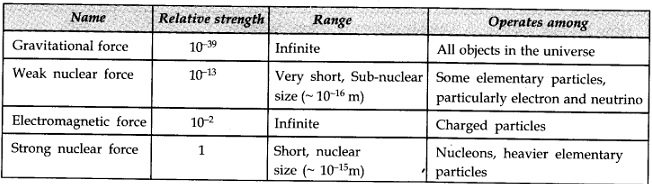There are four fundamental forces in the nature that govern the diverse phenomena of the macroscopic and the microscopic world.

These are the ‘gravitational force‘, the ‘electromagnetic force‘, the ‘strong nuclear force‘, and the ‘weak nuclear force‘.

Unification of different forces/domains in nature is a basic quest in physics.

## Gravitational Force:

The gravitational force is the force of mutual attraction between any two objects by virtue of their masses. It is a universal force.

• Gravity governs the motion of the moon and artificial satellites around the earth, the motion of the earth and other planets around the sun.
• Gravity governs the motion of bodies falling to the earth.
• It plays a key role in the large-scale phenomena of the universe, such as formation and evolution of stars, galaxies and galactic clusters.

## Electromagnetic force:

• The electromagnetic force is the force between charged particles.
• In the simpler case when charges are at rest, this force is given by Coulomb’s law.
• Coulomb’s force between charged particles is attractive for unlike charges and repulsive for like charges.
• The charges in motion produce magnetic effects and a magnetic field gives rise to a force on a moving charge. This force is also an electromagnetic force.
• In general, electric and magnetic effects are inseparable, hence the name electromagnetic force.
• Like the gravitational force, the electromagnetic force acts over large distances and does not need any intervening medium.
• The Range of electromagnetic force is infinite.

Major differences between Gravitational force and Electromagnetic force-

• Gravitational force is always attractive, while electromagnetic force can be attractive or repulsive.
• Gravitational force acts between two masses and the mass comes only in one variety (there is no negative mass), but charge responsible for the electromagnetic force, comes in two varieties: positive and negative charges.
• Since matter is mostly electrically neutral (net charge is zero). Thus, the electric force is largely zero and is not manifested in nature so that gravitational force dominates in terrestrial phenomena.
• The electric force is generally 1036 times stronger than the gravitational force.

## Strong nuclear force:

• The strong nuclear force is attractive in nature.
• The strong nuclear force binds protons and neutrons in a nucleus. This attractive force is responsible to hold the protons in nucleus against the electric repulsion between them. In other words we can say that this force is responsible for the stability of nuclei.
• The strong nuclear force is the strongest in all fundamental forces. It is about 100 times stronger than the electromagnetic force and 1039 times stronger than the gravitational force while it is 1013 times to the ‘Weak Nuclear Force’ in strength.
• Strong nuclear force is a charge-independent force. It acts equally between a proton and a proton, a neutron and a neutron, and a proton and a neutron.
• Its range is extremely small, of about nuclear dimensions (10-15m). Since the range of this force is very small so that the electron does not experience this force.

## Weak nuclear force:

• The weak nuclear force appears only in certain nuclear processes such as the β-decay of a nucleus. In β-decay, the nucleus emits an electron and an uncharged particle called neutrino.
• The weak nuclear force is not as weak as the gravitational force, but much weaker than the strong nuclear and electromagnetic forces.

The range of weak nuclear force is exceedingly small, of the order of 10-16 m.

Table of Fundamental forces of nature